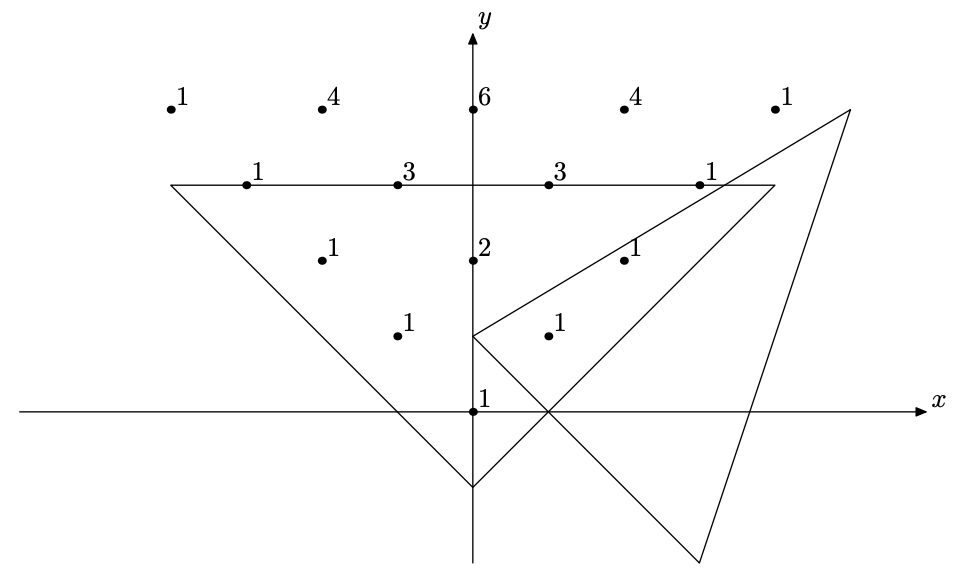시간 제한메모리 제한제출정답맞힌 사람정답 비율
3 초 512 MB167743.750%

## 문제

Grace is developing a brand new theory of geometrical combinatorics --- a study about geometrical properties of combinatoric objects.

Consider two triangles on plane --- a Pascal's triangle and an ordinary triangle. Pascal's triangle is drawn with it's root at point (0, 0), and two sides along diagonals of upper-halfplane quarters. Formally, there are 1's written in points $(i, i)$ and $(-i, i)$, and between them at point $(-i + 2 k, i)$ there is a number equal to the sum of numbers at $(-i + 2k + 1, i - 1)$ and at $(-i + 2k - 1, i - 1)$ for all $k$ from $1$ to $i - 1$. An ordinary triangle is drawn as just a triangle with vertices at $(x_A, y_A)$, $(x_B, y_B)$, $(x_C, y_C)$.Grace defines an intersection value of Pascal's triangle and an ordinary triangle as the sum of values of Pascal's triangle inside or on the border of the ordinary triangle. Can you develop a program that calculates this intersection value?

## 입력

On the first line there is an integer $t$ ($1 \le t \le 5$) --- the number of tests to process. Each of the next $t$ lines contains 6 integers $x_A$, $y_A$, $x_B$, $y_B$, $x_C$, $y_C$ ($-10^6 \le x_A, y_A, x_B, y_B, x_C, y_C \le 10^6$). Three points in each test do not lie on a line.

## 출력

For each test output an integer --- the intersection value modulo $10^9+7$.

## 예제 입력 1

2
0 -1 -4 3 4 3
5 4 0 1 3 -2


## 예제 출력 1

15
2